# I.E. Irodov Solutions on Dispersion and Absorption Of Light

Students can find the solutions to Problems In General Physics I.E. Irodov - Dispersion and Absorption Of Light on this page. In optics, dispersion is an important topic. The main points in this topic include permittivity of substance according to the elementary theory of dispersion, phase velocity v and group velocity u, Rayleigh's formula, attenuation of a narrow beam of electromagnetic radiation, etc. Students are advised to go through these solutions so that they will be familiar with the type of questions asked from optics.

Learning these solutions will help students to easily score higher ranks in the JEE exam and board exams.

### I.E. Irodov Solutions on Dispersion and Absorption Of Light

1. A free electron is located in the field of a monochromatic light wave. The intensity of light is I = 150 W/m2, its frequency is ω = 3.4.1015 s-1. Find:

(a) the electron's oscillation amplitude and its velocity amplitude;

(b) the ratio Fm/Fe, where Fm and Fe are the amplitudes of forces with which the magnetic and electric components of the light wave field act on the electron; demonstrate that that ratio is equal to ½ v/c where v is the electron's velocity amplitude and c is the velocity of light. Instruction. The action of the magnetic field component can be disregarded in the equation of motion of the electron since the calculations show it to be negligible.

Solution:

1. In a travelling plane electromagnetic wave the intensity is simply the time-averaged magnitude of the pointing vector:

I = $\left | \vec{E} \times \vec{H}\right |$

= √(ε00)E2

= cε0E2

On using c = 1/√(ε0μ0)

E√ε0 = H√μ0

(a) Represent the electric field at any point by E = E0 sin ωt. Then for the electron we have the equation

mx = eE0 sin ωt

So x = -(eE0/mω2) sin ωt

The amplitude of the forced oscillation is

eE0/mω2 = (e/mω2) √(2I/cε0)

= 5.1 × 10-16 cm

The velocity amplitude is clearly

eE0/mω = 5.1 × 10-16×3.4 ×1015

= 1.73 cm/sec

(b) For the electric force Fe = amplitude of the electric force

= eE0

For the magnetic force (which we have neglected above), it is

evB = evμ0H

= evE√(ε0μ0)

= evE/c

writing v = -v0 cosωt

where v0 = eE0/mω

we see that the magnetic force is apart from a sign

(ev0E0/2c)sin 2ωt

Hence Fm/Fe = Ratio of amplitudes of the two forces

= v0/2c

= 2.9× 10-11

This is negligible and justifies the neglect of the magnetic field of the electromagnetic wave in calculating v0.

2. Find the free electron concentration in ionosphere if its refractive index is equal to n = 0.90 for radio waves of frequency v = 100 MHz.

Solution:

1. n2 = 1 - n0e202

= 1 - n0e2/4π2ε0mv2

Thus n0 = (4π2v20/e2)(1 - n2)

= 2.36×107 cm-3

3. Assuming electrons of substance to be free when subjected to hard X-rays, determine by what magnitude the refractive index of graphite differs from unity in the case of X-rays whose wavelength in vacuum is equal to λ = 50 pm.

Solution:

1. For hard x- rays, the electrons in graphite will behave as if nearly free and the formula of the previous problem can be applied. Thus

n2 = 1 - n0e202

and n ≅ 1 - n0e2/2ε02

on taking the square root and neglecting higher-order terms.

So (n - 1) = -n0e2/2ε02

= -n0e2λ2/8π2ε0me2

We calculate n0 as follows: There are 6×6.023×1023 electrons in 12 gms of graphite of density 1.6 gm/c.c. Thus n0 = 6 × 6.023 × 1023/(12/1.6) per c.c

Using the values of other constants and λ = 50×10-12 metre we get

n-1 = -5.4×10-7

4. A sounding of dilute plasma by radio waves of various frequencies reveals that radio waves with wavelengths exceeding λ0 = 0.75 m experience total internal reflection. Find the free electron concentration in that plasma.

Solution:

1. In the plasma radio waves with wavelengths exceeding λ0 are not propagated. We interpret this to mean that the permittivity becomes negative for such waves. Thus

0 = 1 - n0e202 If ω = 2πc/λ0

Hence n0e2λ02/4π2ε0mc2 = 1

Or n0 = 4π2ε0mc2/e2λ02

= 1.984×109 per c.c

5. Using the definition of the group velocity u, derive Rayleigh's formula. Demonstrate that in the vicinity of λ = λ' the velocity u is equal to the segment v' cut by the tangent of the curve v (λ) at the point λ' (Fig. below).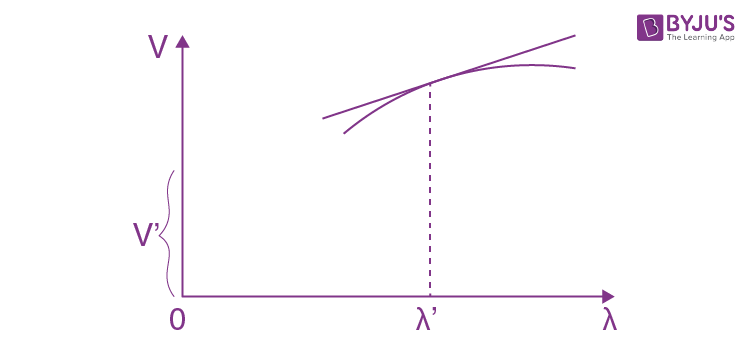Solution:

1. By definition u = dω/dk = (d/dk)(vk)

As ω = vk = v + k dv/dk

Now k = 2π/λ or dk = (-2π/λ2)dλ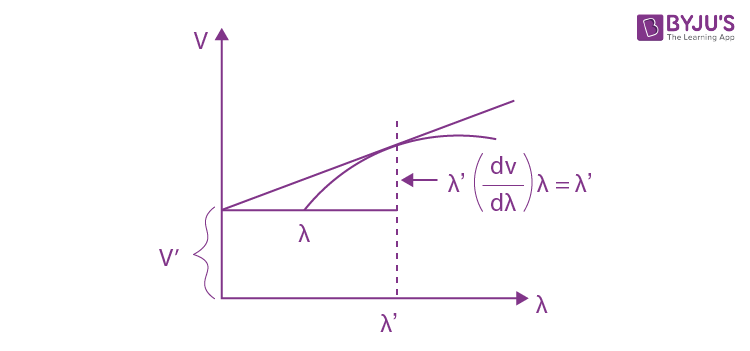Thus u = v - λdv/dλ

Its interpretation is the following

(dv/dλ)λ = λ’ is the slope of the v - λ curve at λ = λ’.

Thus as is obvious from the diagram v’ = v(λ’) - λ’(dv/dλ)λ = λ’ is the group velocity for λ = λ’.

6. Find the relation between the group velocity u and phase velocity v for the following dispersion laws: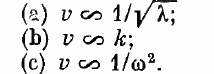Here λ, k, and ω are the wavelength, wave number, and angular frequency.

Solution:

1. (a) v = a/√λ, a = constant

Then u = v - λdv/dλ

= a/√λ - λ(-½ aλ-3/2)

= (3/2).a/√λ

= (3/2) v

(b) v = bk = ωk, b = constant

So ω = bk2 and u = dω/dk = 2bk = 2v

(c) v = c/ω2, c = constant = ω/k

So ω2 = ck or ω = c1/3k/3

Thus u = dω/dk = c1/3(⅓)k-2/3

= ⅓ ω/k

= ⅓ v

7. In a certain medium the relationship between the group and phase velocities of an electromagnetic wave has the form uv = c2, where c is the velocity of light in vacuum. Find the dependence of permittivity of that medium on wave frequency, ε (ω).

Solution:

1. We have uv = (ω/k)dω/dk = c2

Integrating we find ω2 = A + c2k2, A is a constant.

So k = √(ω2 - A)/c

And v = ω/k = c/√(1 - A/ω2)

Writing this as c/√(ε(ω)) we get ε(ω) = 1 - A/ω2

(A can be +ve or negative)

8. A train of plane light waves propagates in the medium where the phase velocity v is a linear function of wavelength: v = a + bλ, where a and b are some positive constants. Demonstrate that in such a medium the shape of an arbitrary train of light waves is restored after the time interval T = 1/b.

Solution:

1. We write v = ω/k = a + bλ

So ω = k(a + bλ) = 2πb + ak

Since k = 2π/λ. Suppose a wavetrain at time t = 0 has the form

F(x, 0) = ∫f(k)eikx dx

Then at time t it will have the form

F(x, t) = ∫f(k)eikx - iωt dk

= ∫f(k)eikx - i(2πb + ak)t dk

= ∫f(k)eik(x - at) e-i2πbt dk

At t = 1/b = T

F(x, T) = F(x - aT, 0)

So at time t = T the wave train has regained its shape though it has advanced by aT.

9. A beam of natural light of intensity I0 falls on a system of two crossed Nicol prisms between which a tube filled with certain solution is placed in a longitudinal magnetic field of strength H. The length of the tube is l, the coefficient of linear absorption of solution is x, and the Verdet constant is V. Find the intensity of light transmitted through that system.

Solution:

1. On passing through the first (polarizer) Nicol the intensity of light becomes ½ I0 because one of the components has been cut off. On passing through the solution the plane of polarization of the light beam will rotate by φ = VlH

And its intensity will also decrease by a factor e-xl. The plane of vibration of the light wave will then make an angle 900 - φ with the principal direction of the analyzer Nicol. Thus by Malus’ law the intensity of light coming out of the second Nicol will be

½ I0 e-xl cos2(900 - φ)

= ½ I0 e-xl sin2 φ

10. A plane monochromatic light wave of intensity l0 falls normally on a plane-parallel plate both of whose surfaces have a reflection coefficient ρ. Taking into account multiple reflections, find the intensity of the transmitted light if

(a) the plate is perfectly transparent, i.e. the absorption is absent;

(b) the coefficient of linear absorption is equal to x, and the plate thickness is d.

Solution:

1. (a) The multiple reflections are shown below. Transmission gives a factor (1 - ρ) while reflections give factors of ρ. Thus the transmitted intensity assuming incoherent light is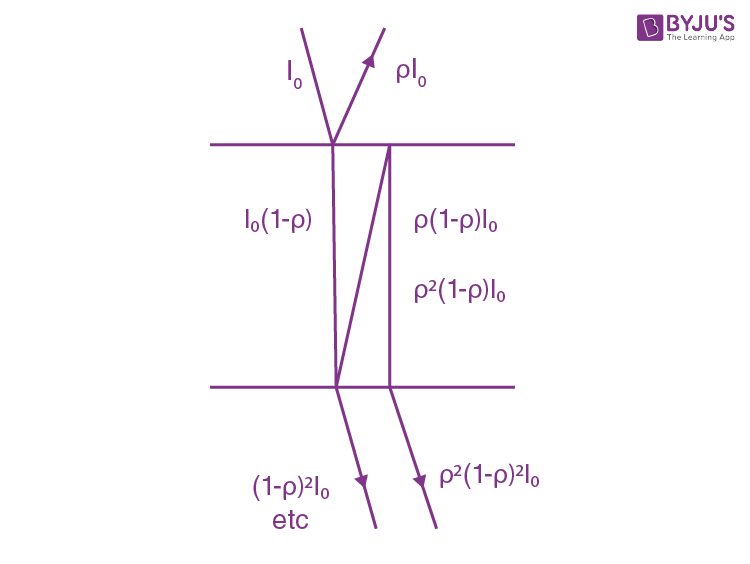(1 - ρ)2I0 + (1 - ρ)2ρ2 I0 + (1 - ρ)2ρ4Io + …

= (1 - ρ)2I0(1 + ρ2 + ρ4 + ρ6 + …)

= (1 - ρ)2I0×1/(1 - ρ2)

= I0(1 - ρ)/(1 + ρ)

(b) When there is absorption, we pick up a factor σ = e-xd in each traversal of the plate. Thus we get

(1 - ρ)2σI0 + (1 - ρ)2σ3ρ2I0 + (1 - ρ)2σ5ρ4I0 +...

= (1 - ρ)2σI0(1 + σ2ρ2 + σ4ρ4 + …)

= I0σ(1 - ρ)2/(1 - σ2ρ2)

11. Two plates, one of thickness d1 = 3 .8 mm and the other of thickness d2 = 9.0 mm, are manufactured from a certain substance. When placed alternately in the way of monochromatic light, the first transmits T1= 0.84 fraction of luminous flux and the second, T2 = 0.70. Find the coefficient of linear absorption of that substance. Light falls at right angles to the plates. The secondary reflections are to be neglected.

Solution:

1. We have

$T_{1}=e^{-\chi d_{1}}(1-\rho )^{2}$

$T_{2}=e^{-\chi d_{2}}(1-\rho )^{2}$

where ρ is the reflectivity; see previous problem, multiple reflection have been ignored.

Thus T1/T2 = ex(d2 - d1)

Or x = ln(T1/T2)/(d2 - d1)

= 0.35 cm-1

12. A beam of monochromatic light passes through a pile of N = 5 identical plane-parallel glass plates each of thickness 1= 0.50 cm. The coefficient of reflection at each surface of the plates is p = 0.050. The ratio of the intensity of light transmitted through the pile of plates to the intensity of incident light is r = 0.55. Neglecting the secondary reflections of light, find the absorption coefficient of the given glass.

Solution:

1. On each surface, we pick up a factor (1 - ρ) from reflection and a factor e-χl due to absorption in each plate.

Thus T = (1 - ρ)2N e-χNl

Thus χ = (1/Nl)ln (1 - ρ)2N/T

= 0.034 cm-1

13. A beam of monochromatic light falls normally on the surface of a plane-parallel plate of thickness l. The absorption coefficient of the substance the plate is made of varies linearly along the normal to its surface from x1 to x2. The coefficient of reflection at each surface of the plate is equal to ρ. Neglecting the secondary reflections, find the transmission coefficient of such a plate.

Solution:

1. Apart from the factor (1 - ρ) on each end face of the plate, we shall get a factor due to absorptions. This factor can be calculated by assuming the plate to consist of a large number of very thin slab within each of which the absorption coefficient can be assumed to be constant Thus we shall get a product like

…..e-χ(x)dx e-χ(x + dx)dx e-χ(x + 2dx)dx …..

This product is nothing but

$e^{-\int_{0}^{l}\chi (x)dx}$

Now χ(0) = χ1, χ(l) = χ2 and variation with x is linear so χ(x) = χ1 + (x/l)(χ2- χ1)

Thus the factor becomes

$-e^{\int_{0}^{l}\left [\chi_{1}+\frac{x}{l}(\chi_{2}-\chi_{1}) dx \right ] }$

= $e^{-\frac{1}{2}(\chi _{1}+\chi _{2})l}$

14. A beam of light of intensity I0 falls normally on a transparent plane-parallel plate of thickness l. The beam contains all the wavelengths in the interval from λ1 to λ2 of equal spectral intensity. Find the intensity of the transmitted beam if in this wavelength interval the absorption coefficient is a linear function of λ, with extreme values x1 and x2. The coefficient of reflection at each surface is equal to ρ. The secondary reflections are to be neglected.

Solution:

1. The spectral density of the incident beam (i.e. intensity of the components whose wavelength lies in the interval λ and λ + dλ) is I0/(λ2 - λ1)dλ, λ1≤ λ≤ λ2

The absorption factor for this component is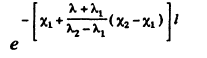And the transmission factor due to reflection at the surface is ( 1 - ρ)2. Thus the intensity of the transmitted beam is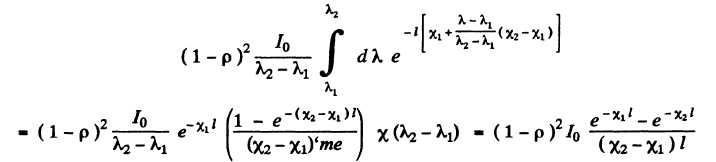15. A light filter is a plate of thickness d whose absorption coefficient depends on wavelength λ as x(λ) = α(1 - λ/λ0)2 cm-1, where α and λ0 are constants. Find the passband λ of this light filter, that is the band at whose edges the attenuation of light is η times that at the wavelength λ0. The coefficient of reflection from the surfaces of the light filter is assumed to be the same at all wavelengths.

Solution:

1. At the wavelength λ0 the absorption coefficient vanishes and loss in transmission is entirely due to reflection. This factor is the same at all wavelengths and therefore cancels out in calculating the pass band and we need not worry about it now

T0 = (transmissivity at λ = λ0) = (1 - ρ)2

T = (transmissivity at λ ) = (1 - ρ)2e-χ(λ)d

The edges of the pass band are λ0 ± ∆λ/2 and at the edge

T/T0 = $e^{-\alpha d(\frac{\Delta \lambda }{2\lambda _{0}})^{2}}$

= η

Thus ∆λ/2λ0 =√((ln 1/η)/αd)

Or ∆λ = 2λ0 √(1/αd(ln 1/η))

16. A point source of monochromatic light emitting a luminous flux Φ is positioned at the centre of a spherical layer of substance. The inside radius of the layer is a, the outside one is b. The coefficient of linear absorption of the substance is equal to x, the reflection coefficient of the surfaces is equal to ρ. Neglecting the secondary reflections, find the intensity of light that passes through that layer.

Solution:

1. We have to derive the law of decrease of intensity in ah absorbing medium taking in to account the natural geometrical fall-off (inverse square law) as well as absorption. Consider a thin spherical shell of thickness dx and internal radius x. Let I(x) and I(x + dx) be the intensities at the inner and outer surfaces of this shell.

Then 4π2I(x) e-x dx = 4π(x + dx)2I(x + dx)2I(x + dx)

Except for the factor e-x dx this is the usual equation. We rewrite this as

x2I(x) = I(x + dx)(x + dx)2(1 + χ dx)

= (I + (dI/dx)dx(x2 + 2x dx) (1 + χ dx)

Or x2dI/dx + χ x2 I + 2xI = 0

Hence d/dx(x2I) + χ(x2I) = 0

So x2I = Ce-χx

Where C is the constant of integration.

In our case we apply this equation for a≤ x ≤ b

For x ≤ a the usual inverse square law gives

I(a) = Φ/4πa2

Hence C = (Φ/4π)eχa

And I(b) = (Φ/4πb2)e-χ(b - a)

This does not take into account reflections. When we do that we get

I(b) = (Φ/4πb2)(1 - ρ)2e-χ(b - a)

17. How many times will the intensity of a narrow X-ray beam of wavelength 20 pm decrease after passing through a lead plate of thickness d = 1.0 mm if the mass absorption coefficient for the given radiation wavelength is equal to μ/ρ = 3.6 cm2/g?

Solution:

1. The transmission factor is e-μd and so the intensity will decrease eμd

= e3.6×11.3×0.1

= 58. 4 times

We have used μ = (μ/ρ)×ρ and used the known value of density of lead.

18. A narrow beam of X-ray radiation of wavelength 62 pm penetrates an aluminium screen 2.6 cm thick. How thick must a lead screen be to attenuate the beam just as much? The mass absorption coefficients of aluminium and lead for this radiation are equal to 3.48 and 72.0 cm2/g respectively.

Solution:

1. We require μpbdpb = μAldAl

Or (μpbpb) ρpbdpb = (μAlAlAldAl

72.0×11.3×dpb = 3.48×2.7×2.6

dpb = 0.3 mm

19. Find the thickness of aluminium layer which reduces by half the intensity of a narrow monochromatic X-ray beam if the corresponding mass absorption coefficient is μ/ρ = 0.32 cm2/g.

Solution:

1. 1/2 = e-μd

Or d = ln 2/μ

= ln 2/(μ/ρ)ρ

= 0.80 cm

20. How many 50%-absorption layers are there in the plate reducing the intensity of a narrow X-ray beam η = 50 times?

Solution:

1. We require N plates

(½)N = 1/50

So N = ln 50/ln 2

= 5.6